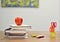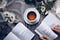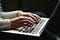This article will outline all of the key functionalities that Pandas library offers. I will demonstrate how powerful the library is and how it can save you time and effort when implementing Python applications.

I have divided this article into three sections.Photo by Element5 Digital on Unsplash

If you want to understand everything about Python programming language, please read:

# Section 1: Pandas Introduction

This section will introduce the readers to the Pandas package and it will also highlight the three most important data structures of the library.

# What is Pandas?

• One of the most widely used Python library for data analysis and engineering.
• Implemented in 2008 by Wes McKinney
• Open source
• Implemented on top of C — hence it’s fast
• Introduced to the DataFrame, Series and Panel objects

# How Do I Install Pandas?

Use Python Package Installer Pip

`pip install pandas`

# What Is A Series?

• Series is 1 dimensional in nature such as an array. Series is a mutable data object. It means it can be updated, new items can be added and existing items can be deleted from the collection.
• It can hold data of any type.
• It can be instantiated with an array or a dictionary. The keys of the dictionary are used to represent indexes.
• The constructor of Series can be called by passing in data, index, data type and a Boolean flag indicating if we want to copy the data. If we don’t specify the index names then the indexes are labeled as integers starting from 0.
• Think of Series as Vertical Columns that can hold multiple rows.

## To Create A Series

`import pandas as pdimport numpy as npseries = pd.Series(data=[111, 222, 3], index = ['one','two','three'])#or evenseries = pd.Series([111, 222, 3])`

## To Retrieve Data From Series

We can apply array splice and index functionality. We can also pass in the index name:

`print(series['one'])orprint(series)#Multiple columnsprint(series[['one','two']]`

# What Is A Data Frame?

• Possibly the most used data structure in a data science project.
• It is a table with rows and columns — like a SQL Table Or Excel Spreadsheet Sheet.
• The table can store in memory data objects in different format.
• Offers high performing time series data analysis and engineering
• A data frame is a container of one or more Series.
• DataFrame is mutable.

This image outlines how a data frame looks:

## Creating A DataFrame

There are several ways of creating a DataFrame; from array, dictionary, list, series or even from another data frame. I usually create a DataFrame from a Series:

`import pandas as pdd = {'ColumnA' : pd.Series([111, 222, 333])   'ColumnB' : pd.Series([444, 555, 666])}df = pd.DataFrame(d)`

The above data frame will contain two columns named ColumnA and ColumnB.

ColumnA will contain three rows: 111,222 and 333.

ColumnB will contain three rows: 444,555 and 666

## Column Selection

We can use the column name to get the data:

`data = df['ColumnA']`

If we want to delete a column then use pop function.

## Row Selection

If a row has a label then we can use loc function:

`data = df.loc['label']#multiple columnsdata = df.loc[['label1', 'label2']]`

Otherwise, we can also use the location by using iloc function:

`data = df.iloc # this will return the first row`

We can also use ix() which let’s us use label and falls back to integer positional access. Note: ix() has been deprecated.

## Deletion

If we want to delete a row then use drop function.

When the data is loaded into a DataFrame then we can apply a number of routines, for example columns can be merged, any number of mathematical calculations can be applied on the data and so on. DataFrame allows us to perform Set based operations.

Each column is indexed.

## Renaming Labels

We can use the rename(columns, index, inplace=False) to rename the columns of a DataFrame. If inplace=True then the underlying data will be renamed. The function does not return a new object. When `inplace=False` is passed (default value) then it performs the operation and returns a copy of the object.

## Reindex

If you want to reindex (change the row/column labels) then we can use the reindex function

## Iterating Over DataFrame Columns

To loop over columns of a data frame, we can do:

`for column in dataFrame:  print(column)`

We can also iterate over the items:

`for column,items in dataFrame.iteritems():`

To iterate over rows:

`for index_of_row, row in dataFrame.iterrows():`

itertuples() is used to display each row as an object:

`for row in dataFrame.itertuples():`

DataFrame is very powerful. We can easily sort items by rows and columns.

## Sorting By Rows:

We can sort by row index by implementing:

`sorted_dataFrame = dataFrame.sort_index()#optionally pass in the sorted row labels`

## Sorting By Columns:

We can utilise sort_index(axis=1) method to sort by columns.

We can also use sort_values(by=’list of columns’) to sort by a number of columns:

`sorted_dataFrame = dataFrame.sort_values(by='ColumnA')`

String based functions can also be applied to columns/rows of a data frame such as lower(), upper(), len(), strip() etc.

# Panel

A panel has three axis. The first axis contains the DataFrames. The second axis is known as the major axis and it is the index of each of the DataFrames. The third axis is the columns of each of the DataFrame.

Note: Panel has been deprecated. Multindex is an alternative data structure.

`import pandas as pdimport numpy as npdata = {'FirstDataFrame' : pd.DataFrame(data),    'SecondDataFrame' : pd.DataFrame(data)}p = pd.Panel(data)`Photo by Thought Catalog on Unsplash

# Section 2: Pandas Functionality — Must Know

This section provides an overview of must know functionality that Pandas offers. It will be apparent to see that most of the common use-cases of data manipulation can be dealt by the basic functionalities which I will outline here:

# Reading CSV File

Let’s start with the most common task of reading a csv file and creating a data frame out of it:

`import pandas as pddataFrame=pd.read_csv("mycsv.csv",index_col=['ColumnA'])`

# Reading Excel File

We can read an excel file into a data frame:

`pd.read_excel('myExcel.xlsx', index_col=['ColumnA'])`

We can also load one sheet into a data frame:

`pd.read_excel(open('myExcel.xlsx', 'rb'), sheet_name='Sheet1')`

Use head(n) to return the first n records

`r = dataFrame.head(10) #will return first 10 records`

## Tail

Use tail(n) to return the last n records

`r = dataFrame.tail(10) #will return last 10 records`

## Transpose

If you want to swap rows and columns, use the T attribute

`transposed = dataFrame.T`

There are also key attributes of a DataFrame such as:

• shape — shows dimensionality of the DataFarme
• size — number of items
• ndim — number of axes

## Describe

If you want to see a quick summary of your data frame and want to be informed of its count, mean, standard deviation, minimum, maximum and a number of percentiles for each of the columns in the data frame then use the describe method:

`dataFrame.describe()`

DataFrame also offers a number of statistic functions such as:

• abs() — Absolute values
• mean() — Mean values. It also offers median(), mode()
• min() — minimum value. It also offers max()
• count(), std() — standard deviation, prod() — to calculate product of the values and cumsum() to calculate cumulative sum etc

Sometimes we want to apply our own custom functions

# Table Function

To apply a custom function on all of the columns of a data table, use the pipe() method:

`def myCustom(a,b): return a-bdataFrame.pipe(myCustom, 1) # last parameter is the value of b in myCustom`

# Row/Column Function

If you want to apply a function to a row or a column then use apply():

This will apply myCustom function to all columns:

`def myCustom(a): return a-1dataFrame.apply(myCustom)`

If you want to apply a function to each row:

set axis=1 e.g.

`dataFrame.apply(myCustom, axis=1)`

If you want to apply a function to each column:

set axis=0 e.g.

`dataFrame.apply(myCustom, axis=0)`

Note: default parameter value of axis is 0

# Element Function

We can use the map() function on Series and applymap() on a DataFrame:

`dataFrame.applymap(myCustom)`Photo by Kaitlyn Baker on Unsplash

# Section 3: Pandas Functionality: Data Engineering

Pandas is a fantastic library when it comes to performing data engineering tasks. This section will provide details of the key features that Pandas provides.

Firstly and most importantly, when working on a data science project, we are often faced with missing data. To fill missing data, we can replace a blank value with a pre-defined value or we can use backward or forward filling.

# To Check For Missing Values

`dataFrame.notnull()`

# To Drop Missing Values

`dataFrame.dropna()`

# Filling Missing Values — Direct Replace

`dataFrame.fillna(ScalarValue)`

We can also pass in a dictionary and use the replace() method to replace the items with the replaced value.

# Filling Missing Values — Backward Or Forward

`dataFrame.fillna(method='backfill') #ffill for forward fill`

# Comparing Elements In Percentage

We can compare elements in a data frame and compute a percentage change of each element with its prior element:

`dataFrame.pct_change() #column wisedataFrame.pct_change(axis=1) #row wise`

# Computing Standard Deviation

We can do std**2 to find variance of each column

`dataFrame.std() #std of each column`

# Computing Covariance

To compute covariance between two columns of a data frame:

`dataFrame.cov() #between all columnsdataFrame['columnA'].cov(dataFrame['columnB']) # between two columns`

# Computing Correlation

`dataFrame.corr() #between all columnsdataFrame['columnA'].corr(dataFrame['columnB']) # between two columns`

# Computing Rolling Moving Average With Window

To compute rolling window average, here average could be any statistical measure for any size window:

`dataFrame.rolling(window=N).median()`

This will then replace the Nth row onwards by the median of the previous N rows.

# Computing Expanding And Exponentially Weighted Average

We can also use expanding() method to perform expanding transformation.

Exponentially weighted average can also be computed by using evm() function:

`dataFrame.ewm(com=0.5).median()`

# Aggregating Columns

A number of functions can be applied to a number of columns in a DataFrame by using the aggregate function:

`dataFrame.aggregate({'ColumnName': function})`

# Grouping Rows

We can use a groupby() function:

`groupedDataFrame = dataFrame.groupby('ColumnName')#multiple grouping columnsgroupedDataFrame = dataFrame.groupby(['ColumnA', 'ColumnB')`

To view the groups:

`groupedDataFrame.groups`

To select a group:

`groupedDataFrame.get_group(key)`

# Filtering

We can execute filter(function) to filter the records:

`dataFrame.filter(myCustomFunction) #myCustomFunction takes in a parameter and returns a value`

# Merging

Joining two data frames is probably one of the most important data science tasks. Pandas offers a range of merging functionality whereby multiple data frames can be joined based on left, right, full inner and outer join.

The function to merge is called merge() that takes in left data frame, right data frame, and on parameter defining which columns we want to join on and how parameter outlining the join e.g. left, right, outer or inner.

Note: If we want to choose different columns on left and right data frame then we can use left_on and right_on parameters and specify the column names. The columns can be a list of columns.

Example:

`merged = pd.merge(left,right,left_on='name',right_on='id', how='left')`

# Union Data Frames

To concatenate two data frames, use concat() function:

`pd.concat([one, two])`

# To Compute Dates

Pandas offers a range of functionality to compute dates in a data frame. We can use:

`pd.date_range(start, end)`

We can also pass in a number of frequencies such as business date, weekly, monthly etc.

# Plotting Data Frame

Data frame offers a range of graphical plotting options. We can plot, box plot, area, scatter plots, stacked charts, bar charts, histograms, etc.

`dataFrame.plot.bar() # creates a bar chartdataFarme.diff.hist(bins=10) # creates a histogramdataFrame.plot.scatter() #plots a scatter chart`

If you want to understand everything about Python programming language, please read:

# Summary

This article outlined and provided an overview of one of the most important python libraries known as Pandas.

It introduced the library and then highlighted all of the key areas of the library.

Please let me know if you have any feedback.

Hope it helps.

Written by